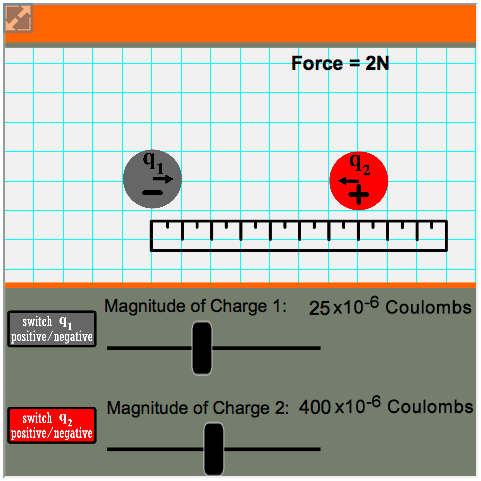###Coulomb's Law

Resource:
Physics Interactives - Coulomb's Law

Law of Electrostatic Force - Student Activity

Description:
This activity is intended for use in the early stages of a learning cycle on Electrostatics and Coulomb’s Law. How is electrostatic force related to magnitude of charge and to separation distance between charged objects? Students explore this question as they change the quantity of charge and amount of separation between two point charges. The simulation can be run alone for an inquiry-based experience, or teachers can introduce the companion Student Exercise for a more guided lesson.

Performance Expectation:
Motion and Stability - Types of Interactions (HS-PS2-4):  Use mathematical representations of Newton’s Law of Gravitation and Coulomb’s Law to describe and predict the gravitational and electrostatic forces between objects.

This activity aligns with the three dimensions of the Next Generation Science Standards in the manner described below:

Disciplinary Core Ideas
 Types of Interactions (HS-PS2.B.1):  Newton’s Law of Universal Gravitation and Coulomb’s Law provide the mathematical models to describe and predict the effects of gravitational and electrostatic forces between distant objects. Many students can do the math involved with Coulomb’s equation, but may have little comprehension of its significance. This simulation lets students visualize charge interaction to see why Coulomb’s Law works mathematically and why this powerful law of physics was essential to development of the theory of electromagnetism. The accompanying Student Exercise provides guided scaffolding for performing the calculations.

Crosscutting Concepts
 Patterns:  Different patterns may be observed at each of the scales at which a system is studied and can provide evidence for causality in explanations of phenomena. In performing this activity, students will be able to see patterns that are not visible on the macroscale. The simulation allows them to switch the sign of the charge (positive/negative), change the quantity of charge on either object, and move the charges around on a grid. Vector arrows show the force interaction. Even before attempting the mathematics of Coulomb’s equation, students will see a pattern emerge that relates force to separation distance and magnitude of charge. Scale, Proportion, and Quantity: The significance of a phenomenon is dependent on the scale, proportion, and quantity at which it occurs. The Student Exercise provides a guided experience for investigating these specific proportional relationships: 1) the direct proportionality between electrostatic force and magnitude of charge, and 2) the inverse relationship between force and separation distance.

Practices
 Analyzing and Interpreting Data:  Analyze data using models (e.g. computational, mathematical) in order to make valid and reliable scientific claims or determine an optimal design solution. The simulation and companion student exercise call for students to collect specific data sets as they run the Coulomb’s Law model, enter the data in tables, and perform calculations to determine mathematical relationships between electrostatic force, quantity of charge, and separation distance between charged objects. Developing and Using Models:  Develop and/or use a model to generate data to support explanations, analyze systems, or solve problems. The Coulomb’s Law interactive simulation allows students to generate specific data to analyze what happens to electrostatic force when magnitude of charge is doubled, tripled, or quadrupled. They also use the model to measure separation distance between two point charges. The model automatically displays the amount of force in Newtons, which the students enter into data tables to analyze the pattern between force and distance of separation. Their final task is to make a scientific claim describing the relationships. Engaging in Argument from Evidence: Make and defend a claim based on evidence about the natural world that reflects scientific knowledge and student-generated evidence. The companion Student Exercise involves two data-gathering tasks performed by using the Coulomb’s Law Interactive model. At the end of each task, students must make scientific claims addressing the following two concepts:  1) the relationship between electrostatic force and magnitude of charge, and 2) the relationship between electrostatic force and separation distance between the charged objects. They must defend their claims with evidence from their own data sets.

Associated Reading from The Physics Classroom

Other Supporting Pages at The Physics Classroom:

View Infographic.
(Coming Soon)

### Search the NGSS Corner

Maybe you're looking for something really specific that pertains to a desired topic and emphasizes one or more of the listed NGSS dimensions. Why not try a search of this section of our website? Simply select from one or more of the pull-down menus and click Search.  This page will reload and a collection  of possibilities will be displayed in this section of the page and sorted by relevancy.